## Least Common Multiple

The least common multiple of two numbersandis denotedorand can be obtained by finding the Prime factorization of each(1)(2)

where thes are all Prime Factors ofand, and ifdoes not occur in one factorization, then the corresponding exponent is 0. The least common multiple is then(3)

Letbe a common multiple ofandso that(4)

Write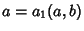and, where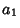and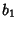are Relatively Prime by definition of the Greatest Common Divisor. Then, and from the Division Lemma (given that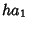is Divisible byand), we have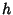is Divisible by, so(5)(6)

The smallestis given by,(7)

so(8)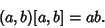(9)

The LCM is Idempotent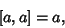(10)

Commutative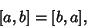(11)

Associative(12)

Distributive(13)

and satisfies the Absorption Law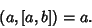(14)

It is also true that(15)

Guy, R. K. Density of a Sequence with L.C.M. of Each Pair Less than.'' §E2 in Unsolved Problems in Number Theory, 2nd ed. New York: Springer-Verlag, pp. 200-201, 1994.